Thread: Eric Dollard View Single PostWeb000xSilver Member Join Date: Apr 2009 Posts: 547
Eric Dollard Inspired Parameter Variation

The following posts are in regard to Eric Dollard inspired parameter variation taken from the Parameter Variation Machines With focus on EPD FQToEW, JF Murray, Manelstam & Papalex thread:

As promised, here is a bit of information about what I have been doing behind the scenes. It has not reached the stage of development that we are all seeking for yet, but it does provide some valuable insights to the beginning experimenter that could help save a lot of time.

First of all, let us examine what direction Eric Dollard has been pointing us towards. Examine the waveforms presented in the following diagram:Notice that the power wave, P, in watts has an unequal amount of area underneath the curve. In the first diagram, the energy is being produced (the negative area under the power wave is greater than the positive area under the power wave) and in the second diagram, energy is being consumed (the positive area under the power wave is greater than the negative area under the power wave). Analyzing the sinusoidal waves in the graph shows that if the circuit did not have the parameter variation denoted by the wave labeled x, it would exhibit an induction factor of 100% and a power factor of 0%, meaning that this circuit would be a purely reactive circuit, a very high if not infinite magnification factor. This graph clearly shows that under certain conditions of synchronous parameter variation that a negative power factor can manifest in a circuit, meaning that energy is being externally supplied from the varying parameter. Where does the energy come from? That is the million dollar question...

As suggested by Eric Dollard, how do we construct a magnetic amplifier circuit to use as a means of synchronous parameter variation at the 2nd harmonic of the power wave? If you just simply connect up a circuit like this, complications will occur.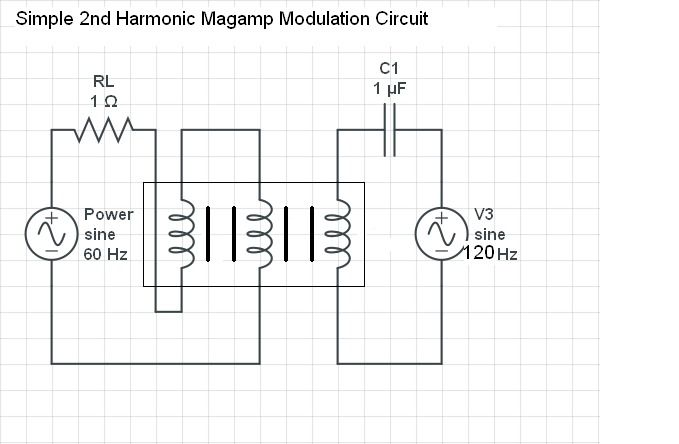The above circuit will have the following waveshapes where the function shown in black is the power wave (carrier wave) and shown in blue is the modulation wave: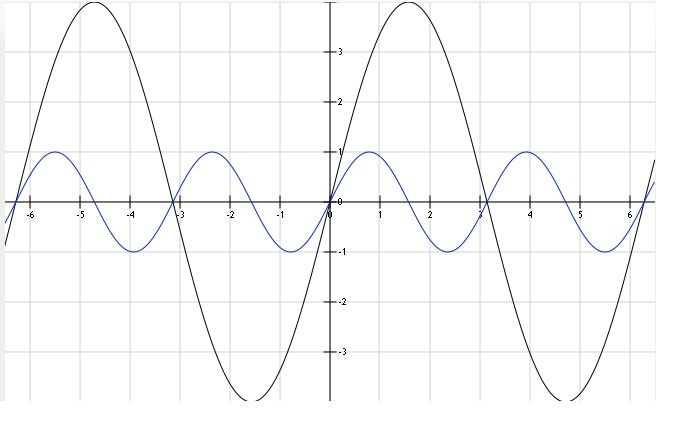The above graph seems like it is suited to be the correct waveshape that we want for our modulation, HOWEVER, if you start taking DC inductance measurements for both polarities of ampere-turns in the control winding, you will see that the inductance of the power windings decreases with each half cycle of the modulation wave, giving 4 minimum inductance peaks rather than 2 as shown by Steinmetz's diagram above. A graphical representation can be seen below, inductance colored red: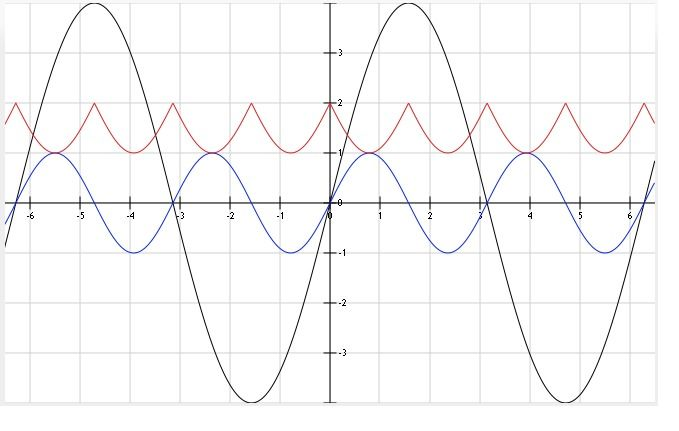As can be seen by the series capacitor in the "Simple Second Harmonic Magamp Modulation Circuit", the modulation portion needs to be in resonance to effectively deliver enough current into the impedance (control winding) that will in turn deliver the ampere-turns of magnetism into the core. After many, many hours of trying every damn thing that I could to overcome this problem of the 4x inductance crests/troughs, I found a simple solution. Two identical control windings and a couple of diodes! So easy...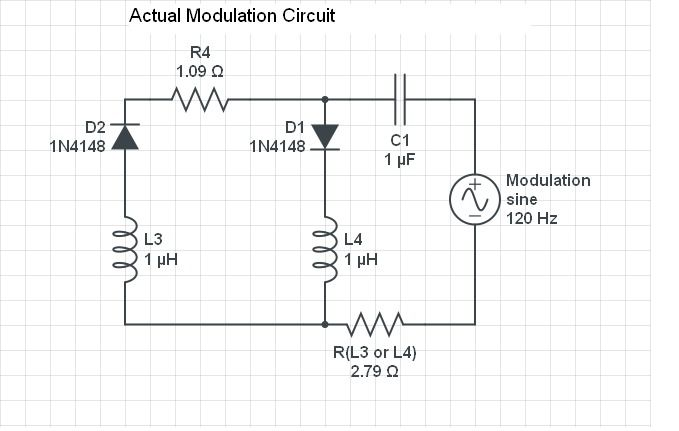*R4 is the 1 ohm resistor for current detection.

This arrangement allows for unidirectional current only in the control winding of the magamp under study. It still doesn't have the sinusoidal shape that Steinmetz's has, but the inductance variation becomes isolated to only two out of the four quadrants of rotation. It has produced the best looking waveforms that I have seen on my scope to date.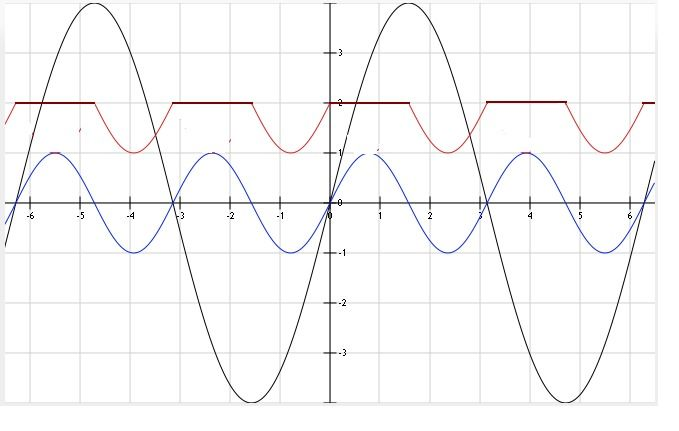This configuration would be best used on a four phase electrical system. Then all diverted control winding current could be utilized to modulate another core, creating a balanced system.

This sums up how I have been running my modulation experiments, and I'll be posting some data to accompany this when I can stand to sit down at the computer again.

Until then...

Dave
__________________